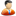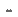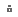﻿ Udemy - The Complete Supervised Machine Learning Models in Python » GFxtraUdemy - The Complete Supervised Machine Learning Models in Python

In this course, you are going to learn all types of Supervised Machine Learning Models implemented in Python. The Math behind every model is very important. Without it, you can never become a Good Data Scientist. That is the reason, I have covered the Math behind every model in the intuition part of each Model.

## Description

In this course, you are going to learn all types of Supervised Machine Learning Models implemented in Python. The Math behind every model is very important. Without it, you can never become a Good Data Scientist. That is the reason, I have covered the Math behind every model in the intuition part of each Model.

Implementation in Python is done in such a way so that not only you learn how to implement a specific Model in Python but you learn how to build real times templates and find the accuracy rate of Models so that you can easily test different models on a specific problem, find the accuracy rates and then choose the one which give you the highest accuracy rate.I am looking forward to see you in the course..

Best

Who this course is for:
• Anyone who wants to learn Supervised Machine Learning Models
• Anyone who wants to learn the Math behind Machine Learning Models
• Anyone curious about Data Science

## Course content

• Introduction
• What is Machine Learning
• Supervised vs Unsupervised Machine Learning Models
• Installing the Spyder IDE
• Data sets for the Course
• Simple Linear Regression Model
• Simple Linear Regression Intuition 1
• Simple Linear Regression Intuition 2
• Simple Linear Regression Intuition 3
• Simple Linear Regression implementation in Python
• Simple Linear Regression Python Part 1
• Simple Linear Regression Python Part 2
• Simple Linear Regression Python Part 3
• Simple Linear Regression Python Part 4
• Simple Linear Regression Python Part 5
• Simple Linear Regression Python Part 6
• Simple Linear Regression Python Part 7
• Multiple Linear Regression Model Intuitions
• Multiple Linear Regression Intuition 1
• Multiple Linear Regression Intuition 2
• Multiple Linear Regression Intuition 3
• Multiple Linear Regression Intuition 4
• Multiple Linear Regression Intuition 5
• Multiple Linear Regression Intuition 6
• Multiple Linear Regression Model implementation in Python
• Multiple Linear Regression Python 1
• Multiple Linear Regression Python 2
• Multiple Linear Regression Python 3
• Multiple Linear Regression Python 4
• Multiple Linear Regression Python 5
• Multiple Linear Regression Python 6
• Multiple Linear Regression Python 7
• Polynomial Regression Model Intuitions
• Polynomial Regression Intuition 1
• Polynomial Regression Model implementation in Python
• Polynomial Regression Python 1
• Polynomial Regression Python 2
• Polynomial Regression Python 3
• Ridge Regression Model Intuitions
• Ridge Regression Intuition 1
• Ridge Regression Intuition 2
• Ridge Regression implementation in Python
• Ridge Regression Python 1
• Ridge Regression Python 2
• Ridge Regression Python 3
• Ridge Regression Python 4
• Lasso Regression Model Intuition
• Lasso Regression Intuition 1
• Lasso Regression implementation in Python
• Lasso Regression Python 1
• Lasso Regression Python 2
• Lasso Regression Python 3
• Lasso Regression Python 4
• Decision Tree Regression Model Intuition
• Decision Tree Regression Intuition 1
• Decision Tree Regression implementation in Python
• Decision Tree Regression Python 1
• Decision Tree Regression Python 2
• Decision Tree Regression Python 3
• Decision Tree Regression Python 4
• Decision Tree Regression Python 5
• Random Forest Regression Model Intuition
• Random Forest Regression Intuition 1
• Random Forest Regression implementation in Python
• Random Forest Regression Python 1
• Random Forest Regression Python 2
• Random Forest Regression Python 3
• K Nearest Neighbors Model
• KNN Intuition
• K Nearest Neighbors implementation in Python
• KNN Python 1
• KNN Python 2
• Logistic Regression Model
• Logistic Regression Intuition
• Logistic Regression implementation in Python
• Logistic Regression Python 1
• Logistic Regression Python 2
• Logistic Regression Python 3
• Logistic Regression Python 4
• Logistic Regression Python 5
• Logistic Regression Python 6
• Decision Tree Classification Model
• Decision Tree Classification Intuition
• Decision Tree Classification Intuition 2
• Decision Tree Classification implementation in Python
• Decision Tree Classification Python 1
• Decision Tree Classification Python 2
• Decision Tree Classification Python 3
• Decision Tree Classification Python 4
• Random Forest Classification Model
• Random Forest Classification Intuition
• Random Forest Classification implementation in Python
• Random Forest Classification Python 1
• Random Forest Classification Python 2
• Random Forest Classification Python 3
• Random Forest Classification Python 4
• Random Forest Regression Python 5
• The Naive Bayes Classification Model
• Naive Bayes Intuition 1
• Naive Bayes Intuition 2
• Naive Bayes implementation in Python
• Naive Bayes Python 1
• Naive Bayes Python 2
• Naive Bayes Python 3
• Naive Bayes Python 4
• Support Vector Classification Model
• Support Vector Classification Intuition
• Support Vector implementation in Python
• Support Vector Classification Python 1
• Support Vector Classification Python 2
• Support Vector Classification Python 3
• Support Vector Classification Python 4
• Support Vector Classification Python 5TO MAC USERS: If RAR password doesn't work, use this archive program:

RAR Expander 0.8.5 Beta 4  and extract password protected files without error.

TO WIN USERS: If RAR password doesn't work, use this archive program:

Latest Winrar  and extract password protected files without error.Gamystyle   |

Information
Members of Guests cannot leave comments.